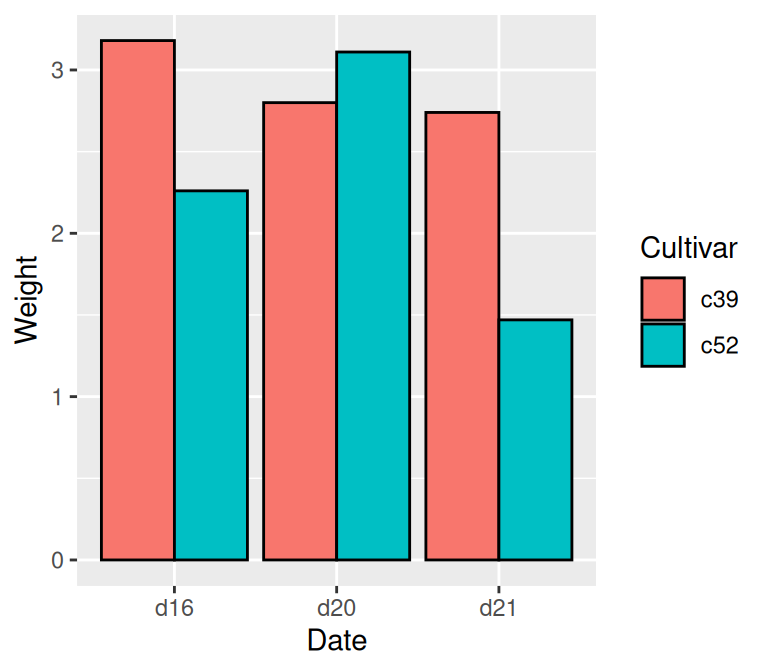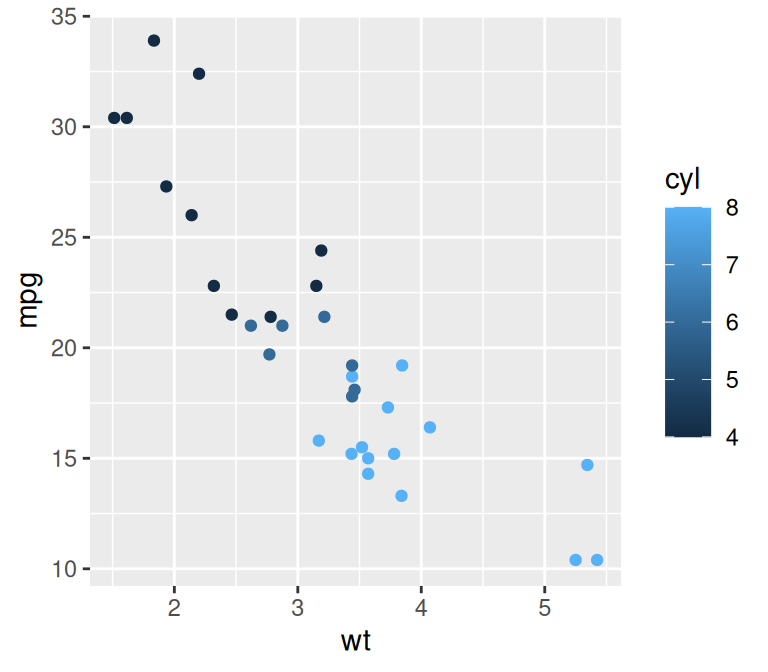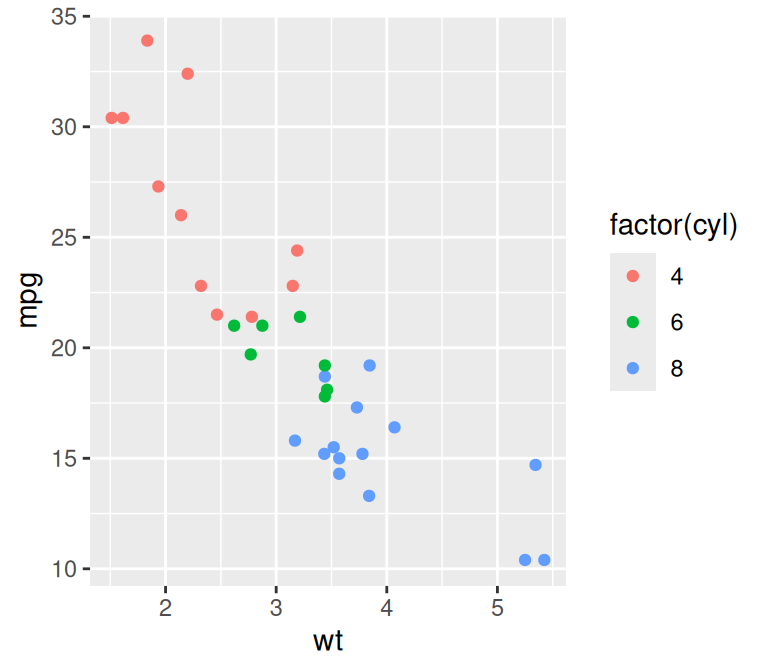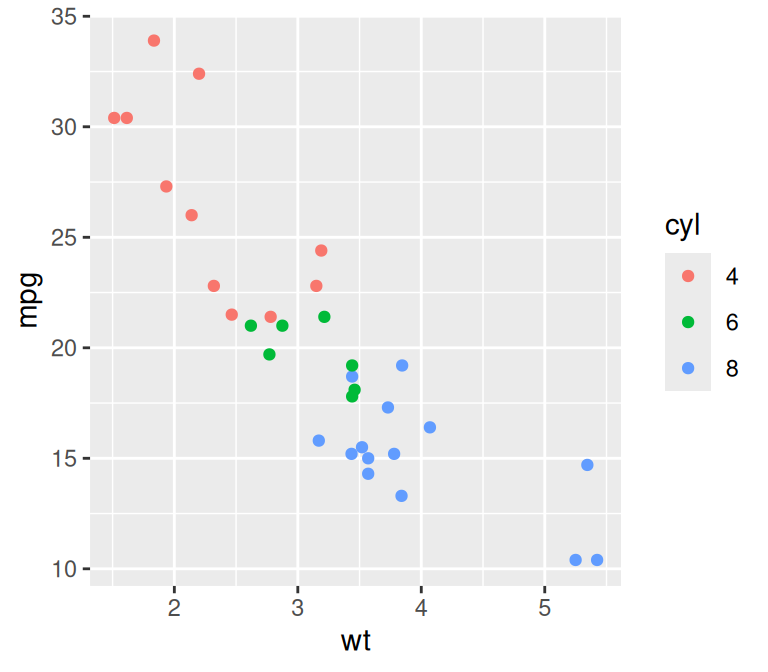## 12.2 Representing Variables with Colors

### 12.2.1 Problem

You want to use a variable (column from a data frame) to control the color of geoms.

### 12.2.2 Solution

In the call to the geom, inside of `aes()`, set the value of `colour` or `fill` to the name of one of the columns in the data (Figure 12.2):

``````library(gcookbook)  # Load gcookbook for the cabbage_exp data set

# These both have the same effect
ggplot(cabbage_exp, aes(x = Date, y = Weight, fill = Cultivar)) +
geom_col(colour = "black", position = "dodge")

ggplot(cabbage_exp, aes(x = Date, y = Weight)) +
geom_col(aes(fill = Cultivar), colour = "black", position = "dodge")

# These both have the same effect
ggplot(mtcars, aes(x = wt, y = mpg, colour = cyl)) +
geom_point()

ggplot(mtcars, aes(x = wt, y = mpg)) +
geom_point(aes(colour = cyl))``````Figure 12.2: Mapping a variable to `fill` (left); Mapping a variable to `colour` for points (right)

When the mapping is specified in `ggplot()` it is used as the default mapping, which is inherited by all the geoms. Within a geom, the default mappings can be overridden.

### 12.2.3 Discussion

In the `cabbage_exp` example, the variable `Cultivar` is mapped to `fill`. The `Cultivar` column in `cabbage_exp` is a factor, so ggplot treats it as a categorical variable. You can check the type using `str()`:

``````str(cabbage_exp)
#> 'data.frame':    6 obs. of  6 variables:
#>  \$ Cultivar: Factor w/ 2 levels "c39","c52": 1 1 1 2 2 2
#>  \$ Date    : Factor w/ 3 levels "d16","d20","d21": 1 2 3 1 2 3
#>  \$ Weight  : num  3.18 2.8 2.74 2.26 3.11 1.47
#>  \$ sd      : num  0.957 0.279 0.983 0.445 0.791 ...
#>  \$ n       : int  10 10 10 10 10 10
#>  \$ se      : num  0.3025 0.0882 0.311 0.1408 0.2501 ...``````

In the `mtcars` example, `cyl` is numeric, so it is treated as a continuous variable. Because of this, even though the actual values of `cyl` include only 4, 6, and 8, the legend has entries for the intermediate values 5 and 7. To make ggplot treat `cyl` as a categorical variable, you can convert it to a factor in the call to `ggplot()` (Figure 12.3, left), or you can modify the data so that the column is a character vector or factor (Figure 12.3, right):

``````# Convert to factor in call to ggplot()
ggplot(mtcars, aes(x = wt, y = mpg, colour = factor(cyl))) +
geom_point()

# Another method: Convert to factor in the data
library(dplyr)
mtcars_mod <- mtcars %>%
mutate(cyl = as.factor(cyl))  # Convert cyl to a factor

ggplot(mtcars_mod, aes(x = wt, y = mpg, colour = cyl)) +
geom_point()``````Figure 12.3: Converting `cyl` to a factor, within the call to ggplot (left); By modifying the dataframe (right)